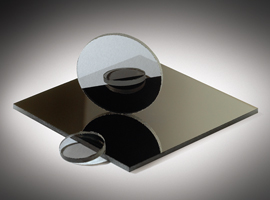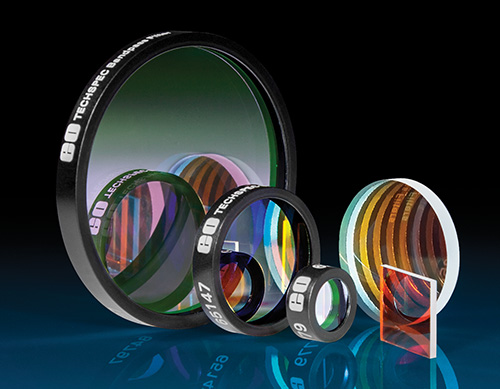# Comprendre les filtres à densité neutreNeutral Density (ND) filters are designed to reduce transmission evenly across a portion of a specific spectrum. ND filters are typically defined by their Optical Density (OD) which describes the amount of energy blocked by the filter. A high optical density value indicates very low transmission, and low optical density indicates high transmission (Equations 1 – 2). ND filters can be stacked to achieve a custom optical density. To calculate the final system OD, simply add the OD of each filter together.

(1)$$T \left(\text{Percent Transmission} \right)=10^{-\text{OD}} \times 100 \%$$

(2)$$\text{OD} = -\log\left(\frac{T}{100 \% }\right)$$

Example 1: What is the transmission if OD 0.3 and OD 1.5 filters are stacked?

(3)$$\text{OD}_{\text{Total}} = 0.3 + 1.5 =1.8$$

(4)$$T = 10^{-1.8} \times 100 \% = 1.58 \%$$

Example 2: How can I build a filter with 0.5% Transmission?

(5)$$\text{OD} = -\log{ \left( \frac{0.5 \% }{100 \%} \right) } = -\log{\left( 0.005 \right)} = 2.3$$

ODTotal of 2.3 could be created by stacking OD 0.3 + OD 2.0 or OD 1.0 + OD 1.3.

## Types of Neutral Density Filters

There are two types of ND filters: reflective and absorptive. Reflective ND filters consist of thin film optical coatings, typically metallic, that have been applied to a glass substrate. The coating can be optimized for specific wavelength ranges such as UV-VIS or NIR. The thin film coating primarily reflects light back toward the source. Special care should be taken to ensure the reflected light does not interfere with the system setup. Absorptive ND filters utilize a glass substrate to absorb light by a specific percentage.Ce contenu vous a-t-il été utile ?